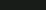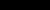# Notes### QFT on curved spacetimes: axiomatic framework and applications

In the spring 2013, at the University of Rome "Tor Vergata", I was giving a course on quantum field theory on curved spacetimes. The lecture notes are available here: Lecture notes (Rome 2013).

For reference on calculus in infinite dimensional topological vector spaces see:
1. Karl-Hermann Neeb, Monastir Lecture Notes on Infinite-Dimensional Lie Groups,
2. Christoph Wockel, Infinite-Dimensional Lie Theory for Gauge Groups,
3. Andreas Kriegl and Peter W. Michor, The Convenient Setting of Global Analysis.### QFT for mathematicians

Between the 6th and 11th of February 2012 I was giving a series of lectures on perturbative algebraic QFT. I combined my lecture notes with the lecture notes of Klaus Fredenhagen from the winter school in Les Houches. They are now available on arXiv: Perturbative algebraic quantum field theory. Here is some further reading, if you are interested in the subject:
1. For introduction to AQFT, see lecture notes of K. Fredenhagen available at: Algebraic Quantum Field Theory.
2. A standard reference on AQFT is also the book of R. Haag, Local quantum physics.
3. For more details on operator algebras you can refer to the book by O. Bratteli and D. W. Robinson Operator Algebras and Quantum Statistical Mechanics, volumes I and II are both available on the author's homepage.
4. A good introduction to methods of functional analysis used in physics is provided in the book of B. Simon and M. Reed, Methods of Modern Mathematical Physics, I: Functional Analysis, Academic Press, 1980. There you can find many examples from physics, including the quantization of the position and momentum of a particle, which I discussed in one of the lectures.
5. Introduction to QFT on curved spacetime is provided in the book of K. Fredenhagen and Ch. Bär, Quantum field theory on curved spacetimes, Lecture Notes in Physics, Vol. 786, Springer Berlin Heidelberg 2009.
6. The standard reference on microlocal analysis (wavefront sets, propagation of singularities, ...) is the book of L. Hörmander The analysis of linear partial differential operators I: Distribution theory and Fourier analysis.
7. To learn more about the spacific applications of microlocal analysis to QFT on curved spacetimes you can look up the following papers:
8. The locally covariant QFT is most exhaustively discussed in the paper of R. Brunetti, K. Fredenhagen and R. Verch, The generally covariant locality principle - A new paradigm for local quantum field theory.
9. Examples of concrete physics applications, which I mentioned briefely during the lecture are available in following references:### Infinite dimnsional differential geometry

In the summer semmester 2009/10 I was organizing in Hamburg a seminar with the title "Infinite dimensional differential geometry". The idea was to learn something more about different approaches to calculus in locally convex vector spaces and apply it to treat for example infinite dimensional Lie groups. The plan of the lectures and some introductary notes are available here. There is also a schedule including the list of topics discussed. Some of the talks are available here:
To learn more about infinite dimensional Lie groups you can look up the webpage of Christoph Wockel and lecture notes available there.